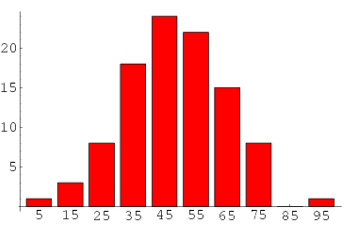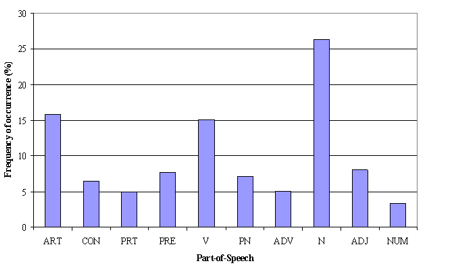# 主题模型 LDA 入门（附 Python 代码）

##### 一、主题模型

• 将“健康”，“医生”，“病人”，“医院” 集合成 “医疗保健” 主题
• 将 “农场”，“玉米”，“小麦” 集合成 “农业”主题

##### 二、狄利克雷分布（Latent Dirichlet Allocation，LDA）主题模型

LDA 模式是生成式模型，在这里，假设需要建模的数据为 X X $X$，标签信息为 $Y$$Y$

LDA 是一种矩阵分解技术，在向量空间中，任何语料（文档的集合）可以表示为文档（Document - Term，DT）矩阵。下面矩阵表达了一个语料库的组成：

. W1 W 1 $W_1$ W2 W 2 $W_2$ Wm W m $W_m$
D1 D 1 $D_1$023
D2 D 2 $D_2$140
... . . . $...$
Dn D n $D_n$110

. Z1 Z 1 $Z_1$ Z2 Z 2 $Z_2$ Zk Z k $Z_k$
θ1 θ 1 $\theta_1$023
θ2 θ 2 $\theta_2$140
... . . . $...$
θn θ n $\theta_n$110

. W1 W 1 $W_1$ W2 W 2 $W_2$ Wm W m $W_m$
ϕ1 ϕ 1 $\phi_1$023
ϕ2 ϕ 2 $\phi_2$140
... . . . $...$
ϕk ϕ k $\phi_k$110

##### LDA 文档生成流程

LDA 假设文档是由多个主题的混合来产生的，每个文档的生成过程如下：

1. 从全局的泊松分布参数为 $\beta$$\beta$ 的分布中生成一个文档的长度 N N $N$
2. 从全局的狄利克雷参数为 $alpha$$alpha$ 的分布中生成一个当前文档的 θ θ $\theta$

• 对当前文档长度 N N $N$ 的每一个字都有
• $\theta$$\theta$ 为参数的多项式分布生成一个主题的下标 zn z n $z_n$
• θ θ $\theta$ z z $z$ 共同为参数的多项式分布中，产生一个字 ${w}_{n}$$w_n$
• 这些主题基于词的概率分布来产生词，给定文档数据集，LDA 可以学习出，是哪些主题产生了这些文档。

对于文档生成过程，则有，首先对于文档 n n $n$ 中的每一个字，都先从文档矩阵 ${M}_{1}$$M_1$ 中的 θi θ i $\theta_i$ 中产生一个下标，告诉我们现在要从主题矩阵 M2 M 2 $M_2$ 中的哪一行 ϕm ϕ m $\phi_m$生成当前的字。

###### 训练过程（吉布斯采样）

吉布斯采样 (Gibbs Sampling) 首先选取概率向量的一个维度，给定其他维度的变量值当前维度的值，不断收敛来输出待估计的参数。具体地

1. 随机给每一篇文档的每一个词 w w $w$，随机分配主题编号 $z$$z$
2. 统计每个主题 zi z i $z_i$ 下出现字 w w $w$ 的数量，以及每个文档 $n$$n$ 中出现主题 zi z i $z_i$ 中的词 w w $w$的数量
3. 每次排除当前词 w w $w$ 的主题分布 ${z}_{i}$$z_i$，根据其他所有词的主题分类，来估计当前词 w w $w$ 分配到各个主题 ${z}_{1},{z}_{2},\dots ,{z}_{k}$$z_1, z_2, …,z_k$ 的概率，即计算 p(zi|zi,d,w) p ( z i | z − i , d , w ) $p(z_i | z_{-i}, d, w )$ (Gibbs updating rule))。得到当前词属于所有主题 z1,z2,,zk z 1 , z 2 , … , z k $z_1, z_2, …, z_k$ 的概率分布后，重新为词采样一个新的主题 z1 z 1 $z_1$。用同样的方法不断更新的下一个词的主题，直到每个文档下的主题分布 θn θ n $\theta_n$ 和每个主题下的词分布 ϕk ϕ k $\phi_k$ 收敛。
4. 最后输出待估计参数， θn θ n $\theta_n$ ϕk ϕ k $\phi_k$ ，每个单词的主题 zn,k z n , k $z_{n,k}$ 也可以得到。

LDA 对于每个文档的每一个字都有一个主题下标。但从文档聚类的角度来说，LDA 没有一个文档统一的聚类标签，而是每个字都有一个聚类标签，这个就是主题。LDA 每个字都有可能属于不同的类别，每个文档都有可能属于不同的类别。在大量的迭代后，主题分布和字分布都比较稳定也比较好了，LDA 模型收敛。

##### 三、LDA 的参数

α α $\alpha$ ：表示 document-topic 密度， α α $\alpha$ 越高，文档包含的主题更多，反之包含的主题更少

β β $\beta$ ：表示 topic-word 密度， β β $\beta$ 越高，主题包含的单词更多，反之包含的单词更少

主题数量：主题数量从语料中抽取得到，使用 Kullback Leibler Divergence Score 可以获取最好的主题数量。

主题词数：组成一个主题所需要的词的数量。这些词的数量通常根据需求得到，如果说需求是抽取特征或者关键词，那么主题词数比较少，如果是抽取概念或者论点，那么主题词数比较多。

迭代次数：使得 LDA 算法收敛的最大迭代次数

##### 四、Running in Python
###### 准备文档集合
doc1 = "Sugar is bad to consume. My sister likes to have sugar, but not my father."
doc2 = "My father spends a lot of time driving my sister around to dance practice."
doc3 = "Doctors suggest that driving may cause increased stress and blood pressure."
doc4 = "Sometimes I feel pressure to perform well at school, but my father never seems to drive my sister to do better."
doc5 = "Health experts say that Sugar is not good for your lifestyle."

# 整合文档数据
doc_complete = [doc1, doc2, doc3, doc4, doc5]

###### 数据清洗和预处理

数据清洗对于任何文本挖掘任务来说都非常重要，在这个任务中，移除标点符号，停用词和标准化语料库（Lemmatizer，对于英文，将词归元）。

from nltk import stopwords
from nltk.stem.wordnet import WordNetLemmatizer
import string

stop = set(stopwords.words('english'))
exclude = set(string.punctuation)
lemma = WordNetLemmatizer()

def clean(doc):
stop_free = " ".join([i for i in doc.lower().split() if i not in stop])
punc_free = ''.join(ch for ch in stop_free if ch not in exclude)
normalized = " ".join(lemma.lemmatize(word) for word in punc_free.split())
return normalized

doc_clean = [clean(doc).split() for doc in doc_complete]

###### 准备 Document - Term 矩阵

语料是由所有的文档组成的，要运行数学模型，将语料转化为矩阵来表达是比较好的方式。LDA 模型在整个 DT 矩阵中寻找重复的词语模式。Python 提供了许多很好的库来进行文本挖掘任务，“genism” 是处理文本数据比较好的库。下面的代码掩饰如何转换语料为 Document - Term 矩阵：

import genism
from gensim import corpora

# 创建语料的词语词典，每个单独的词语都会被赋予一个索引
dictionary = corpora.Dictionary(doc_clean)

# 使用上面的词典，将转换文档列表（语料）变成 DT 矩阵
doc_term_matrix = [dictionary.doc2bow(doc) for doc in doc_clean]

###### 构建 LDA 模型

创建一个 LDA 对象，使用 DT 矩阵进行训练。训练需要上面的一些超参数，gensim 模块允许 LDA 模型从训练语料中进行估计，并且从新的文档中获得对主题分布的推断。

# 使用 gensim 来创建 LDA 模型对象
Lda = genism.models.ldamodel.LdaModel

# 在 DT 矩阵上运行和训练 LDA 模型
ldamodel = Lda(doc_term_matrix, num_topics=3, id2word = dictionary, passes=50)

###### 结果
# 输出结果
print(ldamodel.print_topics(num_topics=3, num_words=3))

[
'0.061*consume + 0.050*drive + 0.050*sister,
'0.049*pressur + 0.049*father + 0.049*sister
]

每一行包含了主题词和主题词的权重，Topic 1 可以看作为“不良健康习惯”，Topic 3 可以看作 “家庭”。

##### 五、提高主题模型结果的一些方法

主题模型的结果完全取决于特征在语料库中的表示，但是语料通常表示为比较稀疏的文档矩阵，因此减少矩阵的维度可以提升主题模型的结果。

###### 1. 根据词频调整稀疏矩阵

根据频率来分布词，高频词更可能出现在结果中，低频词实际上是语料库中的弱特征，对于词频进行分析，可以决定什么频率的值应该被视为阈值。###### 2. 根据词性标注 (Part of Speech Tag) 调整稀疏矩阵

比起频率特征，词性特征更关注于上下文的信息。主题模型尝试去映射相近的词作为主题，但是每个词在上下文上有可能重要性不同，比如说介词 “IN” 包含 “within”，“upon”, “except”，基数词 “CD” 包含：许多(many)，若干（several)，个把(a，few)等等，情态助动词 “MD” 包含 “may”，“must” 等等，这些词可能只是语言的支撑词，对实际意义影响不大，因此可以通过词性来消除这些词的影响。###### 3. 调整 LDA 的 Batch 大小

为了得到主题中最重要的主题词，语料可以被分为固定大小的 batch，在这些 batch 上运行 LDA 模型会提供不同的结果，但是最佳的主题词会在这些 batch 上有交集。

##### 主题模型用于特征选择

比如说文本分类任务中，LDA 可以用来选择特征，因为训练数据中含有类别信息，可以在不同类别的结果中，删除相同的、比较常见的主题词，为主题类别提供更好的特征。

##### 结语

本文主要参考了，没有什么公式，用于对 LDA 有一个大概的了解，后面也会更深入 LDA 模型，可以一边运行上面的代码一边感受 LDA 的作用。

###### 参考文献

09-09
07-21473
11-22
01-0912万+
11-17550
05-316万+
03-152775
04-09294
09-0325万+
05-091万+
03-22
05-151734
04-271万+
07-183359
01-01786
03-073万+
03-221237

### “相关推荐”对你有帮助么？

•非常没帮助
•没帮助
•一般
•有帮助
•非常有帮助被折叠的  条评论 为什么被折叠?到【灌水乐园】发言¥1 ¥2 ¥4 ¥6 ¥10 ¥20获取中扫码支付点击重新获取扫码支付1.余额是钱包充值的虚拟货币，按照1:1的比例进行支付金额的抵扣。
2.余额无法直接购买下载，可以购买VIP、付费专栏及课程。余额充值